Next: 2.4 Time Dependent Problems Up: 2. Finite Element Method Previous: 2.2 Rayleigh-Ritz Method

# 2.3 Galerkin's Method

A weak formulation of () for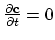is given by,(2.7)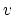is called test function. In this case we have an inner product between a scalar and a vector function on both sides of equation (2.7).

If the solution of the problem (2.1) in the space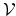exists, than it is possible to represent this solution as the sum of an infinite series with the weighted basis functions of the space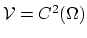.

The central idea of Galerkin's method is to seek the solution of the problem given by (2.1), (2.2), and (2.3) not represented through the basis functions of the infinite spacebut through the basis functions of the finite space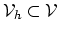( dim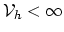) [9,10]. Such a solution can only be an approximation of the real solution of (2.1).
We choose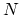linear independent functions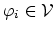,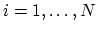which span the space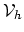, e.g.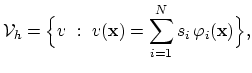(2.8)

where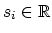.
Now we can write equation (2.7) in the space,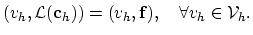(2.9)

Trivially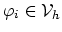, and we can write,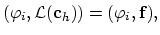for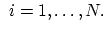(2.10)

Considering that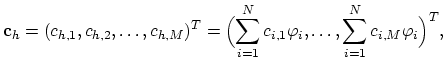(2.11)

we see that inner product in (2.10) transforms the differential operator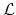into the operator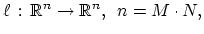(2.12)

and we write (2.10) as a system of nonlinear algebraic equations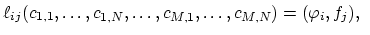for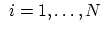and(2.13)

This equation is solved by means of Newton's method (see Section 2.6). The solutions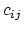of (2.13) are used to construct approximation of the functions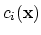fromusing (2.8).
The value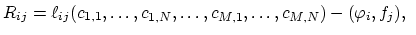forand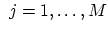(2.14)

is called residuum.Next: 2.4 Time Dependent Problems Up: 2. Finite Element Method Previous: 2.2 Rayleigh-Ritz Method

H. Ceric: Numerical Techniques in Modern TCAD